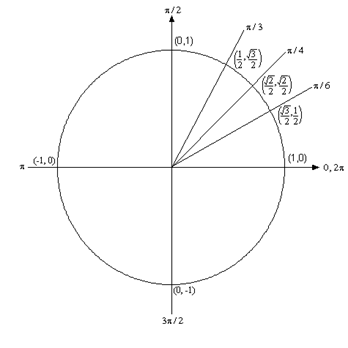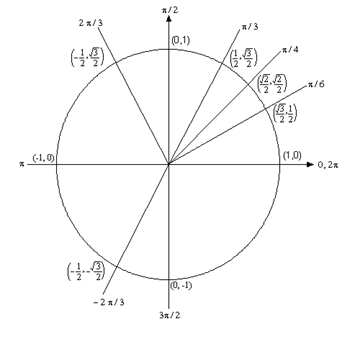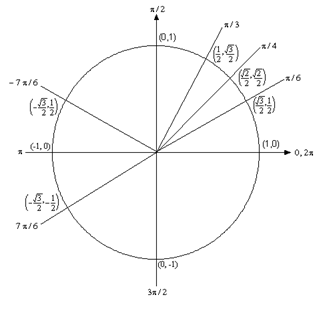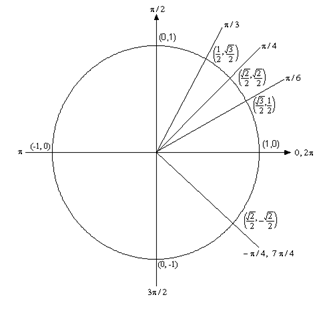Paul's Online Notes
Home / Algebra Trig Review / Trigonometry / Trig Function Evaluation
Show Mobile Notice Show All Notes Hide All Notes
Mobile Notice
You appear to be on a device with a "narrow" screen width (i.e. you are probably on a mobile phone). Due to the nature of the mathematics on this site it is best views in landscape mode. If your device is not in landscape mode many of the equations will run off the side of your device (should be able to scroll to see them) and some of the menu items will be cut off due to the narrow screen width.

### Section 2.1 : Trig Function Evaluation

One of the problems with most trig classes is that they tend to concentrate on right triangle trig and do everything in terms of degrees. Then you get to a calculus course where almost everything is done in radians and the unit circle is a very useful tool.

So first off let’s look at the following table to relate degrees and radians.

 Degree 0 30 45 60 90 180 270 360 Radians 0 $$\displaystyle \frac{\pi }{6}$$ $$\displaystyle \frac{\pi }{4}$$ $$\displaystyle \frac{\pi }{3}$$ $$\displaystyle \frac{\pi }{2}$$ $$\displaystyle \pi$$ $$\displaystyle \frac{{3\pi }}{2}$$ $$\displaystyle 2\pi$$

Know this table! There are, of course, many other angles in radians that we’ll see during this class, but most will relate back to these few angles. So, if you can deal with these angles you will be able to deal with most of the others.

Be forewarned, everything in most calculus classes will be done in radians!

Now, let’s look at the unit circle. Below is the unit circle with just the first quadrant filled in. The way the unit circle works is to draw a line from the center of the circle outwards corresponding to a given angle. Then look at the coordinates of the point where the line and the circle intersect. The first coordinate is the cosine of that angle and the second coordinate is the sine of that angle. There are a couple of basic angles that are commonly used. These are $$0,\frac{\pi }{6},\frac{\pi }{4},\frac{\pi }{3},\frac{\pi }{2},\pi ,\frac{{3\pi }}{2}$$ and $$2\pi$$ and are shown below along with the coordinates of the intersections. So, from the unit circle below we can see that $$\cos \left( {\frac{\pi }{6}} \right) = \frac{{\sqrt 3 }}{2}$$ and $$\sin \left( {\frac{\pi }{6}} \right) = \frac{1}{2}$$.Remember how the signs of angles work. If you rotate in a counter clockwise direction the angle is positive and if you rotate in a clockwise direction the angle is negative.

Recall as well that one complete revolution is $$2\pi$$, so the positive $$x$$-axis can correspond to either an angle of 0 or $$2\pi$$ (or $$4\pi$$, or $$6\pi$$, or $$- 2\pi$$, or $$- 4\pi$$, etc. depending on the direction of rotation). Likewise, the angle $$\frac{\pi }{6}$$ (to pick an angle completely at random) can also be any of the following angles:

$$\displaystyle \frac{\pi }{6} + 2\pi = \frac{{13\pi }}{6}$$ (start at $$\displaystyle \frac{\pi }{6}$$ then rotate once around counter clockwise)

$$\displaystyle \frac{\pi }{6} + 4\pi = \frac{{25\pi }}{6}$$ (start at $$\displaystyle \frac{\pi }{6}$$ then rotate around twice counter clockwise)

$$\displaystyle \frac{\pi }{6} - 2\pi = - \frac{{11\pi }}{6}$$ (start at $$\displaystyle \frac{\pi }{6}$$ then rotate once around clockwise)

$$\displaystyle \frac{\pi }{6} - 4\pi = - \frac{{23\pi }}{6}$$ (start at $$\displaystyle \frac{\pi }{6}$$ then rotate around twice clockwise)

etc.

In fact, $$\frac{\pi }{6}$$ can be any of the following angles $$\frac{\pi }{6} + 2\pi \,n\,,\;\;n = 0,\, \pm 1,\, \pm 2,\, \pm 3,\, \ldots$$ In this case $$n$$ is the number of complete revolutions you make around the unit circle starting at $$\frac{\pi }{6}$$. Positive values of $$n$$ correspond to counter clockwise rotations and negative values of $$n$$ correspond to clockwise rotations.

So, why did I only put in the first quadrant? The answer is simple. If you know the first quadrant then you can get all the other quadrants from the first. You’ll see this in the following examples.

Find the exact value of each of the following. In other words, don’t use a calculator. Show All SolutionsHide All Solutions

1. $$\sin \left( {\frac{{2\pi }}{3}} \right)$$ and $$\sin \left( { - \frac{{2\pi }}{3}} \right)$$
Show Solution

The first evaluation here uses the angle $$\frac{{2\pi }}{3}$$. Notice that $$\frac{{2\pi }}{3} = \pi - \frac{\pi }{3}$$. So $$\frac{{2\pi }}{3}$$ is found by rotating up $$\frac{\pi }{3}$$ from the negative $$x$$-axis. This means that the line for $$\frac{{2\pi }}{3}$$ will be a mirror image of the line for $$\frac{\pi }{3}$$ only in the second quadrant. The coordinates for $$\frac{{2\pi }}{3}$$ will be the coordinates for $$\frac{\pi }{3}$$ except the $$x$$ coordinate will be negative.

Likewise, for $$- \frac{{2\pi }}{3}$$we can notice that $$- \frac{{2\pi }}{3} = - \pi + \frac{\pi }{3}$$, so this angle can be found by rotating down $$\frac{\pi }{3}$$ from the negative $$x$$-axis. This means that the line for $$- \frac{{2\pi }}{3}$$ will be a mirror image of the line for $$\frac{\pi }{3}$$ only in the third quadrant and the coordinates will be the same as the coordinates for $$\frac{\pi }{3}$$ except both will be negative.

Both of these angles along with their coordinates are shown on the following unit circle.From this unit circle we can see that $$\sin \left( {\frac{{2\pi }}{3}} \right) = \frac{{\sqrt 3 }}{2}$$and $$\sin \left( { - \frac{{2\pi }}{3}} \right) = - \frac{{\sqrt 3 }}{2}$$.

This leads to a nice fact about the sine function. The sine function is called an odd function and so for ANY angle we have

$\sin \left( { - \theta } \right) = - \sin \left( \theta \right)$
2. $$\cos \left( {\frac{{7\pi }}{6}} \right)$$ and $$\cos \left( { - \frac{{7\pi }}{6}} \right)$$
Show Solution

For this example, notice that $$\frac{{7\pi }}{6} = \pi + \frac{\pi }{6}$$ so this means we would rotate down $$\frac{\pi }{6}$$ from the negative $$x$$-axis to get to this angle. Also $$- \frac{{7\pi }}{6} = - \pi - \frac{\pi }{6}$$ so this means we would rotate up $$\frac{\pi }{6}$$ from the negative $$x$$-axis to get to this angle. These are both shown on the following unit circle along with appropriate coordinates for the intersection points.From this unit circle we can see that $$\cos \left( {\frac{{7\pi }}{6}} \right) = - \frac{{\sqrt 3 }}{2}$$and $$\cos \left( { - \frac{{7\pi }}{6}} \right) = - \frac{{\sqrt 3 }}{2}$$. In this case the cosine function is called an even function and so for ANY angle we have

$\cos \left( { - \theta } \right) = \cos \left( \theta \right)$
3. $$\tan \left( { - \frac{\pi }{4}} \right)$$ and $$\tan \left( {\frac{{7\pi }}{4}} \right)$$
Show Solution

Here we should note that $$\frac{{7\pi }}{4} = 2\pi - \frac{\pi }{4}$$ so $$\frac{{7\pi }}{4}$$ and $$- \frac{\pi }{4}$$ are in fact the same angle! The unit circle for this angle isNow, if we remember that $$\tan \left( x \right) = \frac{{\sin \left( x \right)}}{{\cos \left( x \right)}}$$ we can use the unit circle to find the values the tangent function. So,

$\tan \left( \frac{7\pi }{4} \right)=\tan \left( -\frac{\pi }{4} \right)=\frac{\sin \left( -{\pi }/{4}\; \right)}{\cos \left( -{\pi }/{4}\; \right)}=\frac{-{\sqrt{2}}/{2}\;}{{\sqrt{2}}/{2}\;}=-1$

On a side note, notice that $$\tan \left( {\frac{\pi }{4}} \right) = 1$$ and we se can see that the tangent function is also called an odd function and so for ANY angle we will have

$\tan \left( { - \theta } \right) = - \tan \left( \theta \right)$
4. $$\sin \left( {\frac{{9\pi }}{4}} \right)$$
Show Solution

For this problem let’s notice that $$\frac{{9\pi }}{4} = 2\pi + \frac{\pi }{4}$$. Now, recall that one complete revolution is $$2\pi$$. So, this means that $$\frac{{9\pi }}{4}$$ and $$\frac{\pi }{4}$$ are at the same point on the unit circle. Therefore,

$\sin \left( {\frac{{9\pi }}{4}} \right) = \sin \left( {2\pi + \frac{\pi }{4}} \right) = \sin \left( {\frac{\pi }{4}} \right) = \frac{{\sqrt 2 }}{2}$

This leads us to a very nice fact about the sine function. The sine function is an example of a periodic function. Periodic function are functions that will repeat themselves over and over. The “distance” that you need to move to the right or left before the function starts repeating itself is called the period of the function.

In the case of sine the period is $$2\pi$$. This means the sine function will repeat itself every $$2\pi$$. This leads to a nice formula for the sine function.

$\sin \left( {x + 2\pi n} \right) = \sin \left( x \right)\hspace{0.25in}\,\,\,\,\,\,\,\,n = 0,\, \pm 1,\,\, \pm 2,\, \ldots$

Notice as well that because

$csc \left( x \right) = \frac{1}{{\sin \left( x \right)}}$

we can say the same thing about cosecant.

$\csc \left( {x + 2\pi n} \right) = \csc \left( x \right)\hspace{0.25in}\,\,\,\,\,\,\,\,n = 0,\, \pm 1,\,\, \pm 2,\, \ldots$

Well, actually we should be careful here. We can say this provided $$x \ne n\pi$$ since sine will be zero at these points and so cosecant won’t exist there!

5. $$\sec \left( {\frac{{25\pi }}{6}} \right)$$
Show Solution

Here we need to notice that $$\frac{{25\pi }}{6} = 4\pi + \frac{\pi }{6}$$. In other words, we’ve started at $$\frac{\pi }{6}$$ and rotated around twice to end back up at the same point on the unit circle. This means that

$\sec \left( {\frac{{25\pi }}{6}} \right) = \sec \left( {4\pi + \frac{\pi }{6}} \right) = \sec \left( {\frac{\pi }{6}} \right)$

Now, let’s also not get excited about the secant here. Just recall that

$\sec \left( x \right) = \frac{1}{{\cos \left( x \right)}}$

and so all we need to do here is evaluate a cosine! Therefore,

$\sec \left( \frac{25\pi }{6} \right)=\sec \left( \frac{\pi }{6} \right)=\frac{1}{\cos \left( \frac{\pi }{6} \right)}=\frac{1}{{}^{\sqrt{3}}/{}_{2}}=\frac{2}{\sqrt{3}}$

We should also note that cosine and secant are periodic functions with a period of $$2\pi$$. So,

\begin{align*}\begin{aligned}\cos \left( {x + 2\pi n} \right) & = \cos \left( x \right)\\ \sec \left( {x + 2\pi n} \right) & = \sec \left( x \right) \end{aligned}& \hspace{0.5in}n = 0, \pm 1, \pm 2,\, \ldots \end{align*}
6. $$\tan \left( {\frac{{4\pi }}{3}} \right)$$
Show Solution

To do this problem it will help to know that tangent (and hence cotangent) is also a periodic function, but unlike sine and cosine it has a period of $$\pi$$.

\begin{align*}\begin{aligned}\tan \left( {x + \pi n} \right) & = \tan \left( x \right)\\ \cot \left( {x + \pi n} \right) & = \cot \left( x \right) \end{aligned}& \hspace{0.5in}n = 0, \pm 1, \pm 2,\, \ldots \end{align*}

So, to do this problem let’s note that $$\frac{{4\pi }}{3} = \pi + \frac{\pi }{3}$$. Therefore,

$\tan \left( {\frac{{4\pi }}{3}} \right) = \tan \left( {\pi + \frac{\pi }{3}} \right) = \tan \left( {\frac{\pi }{3}} \right) = \sqrt 3$

#### Trig Evaluation Final Thoughts

As we saw in the previous examples if you know the first quadrant of the unit circle you can find the value of ANY trig function (not just sine and cosine) for ANY angle that can be related back to one of those shown in the first quadrant. This is a nice idea to remember as it means that you only need to memorize the first quadrant and how to get the angles in the remaining three quadrants!

In these problems I used only “basic” angles, but many of the ideas here can also be applied to angles other than these “basic” angles as we’ll see in Solving Trig Equations.